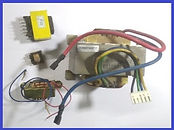top of page

## Transformers

A transformer works on mutual inductance using AC (Alternating Current) power supply as input to step-up or step-down the output voltage.  The input and output coils for mutual inductance are normally connected by a soft iron core.## Basics of Transformer:

A transformer mainly consists of a core made up of steel laminations for magnetic connection between two coils.  Where as, a coil is considered as multiple insulated conducting wires (generally made of copper) wound on the ferrous core. Out of two coils, the coil, which is connected to input AC power supply is called as Primary coil and the other coil, which is generating the AC power due to mutual inductance is called Secondary Coil.

Transformers are available with various types of constructions.  Various shapes of ferrous cores are designed for least leakage of magnetic flux. The ferrous core is generally made of laminated sheets with insulation in between them to have least eddy current losses.  Ferrite core is used for high permeability, which is required, when the frequency of AC input power supply is high. Some times both primary and secondary coils are wound one on another with high electrical insulation in between them.

## Basic Transformer Formulae:For any transformer, the total power conversion is constant, which mainly depends on the primary coil, secondary coil and core.

So, theoretically, Input Power = Output Power, ignoring winding losses, eddy current losses etc.

i.e., Vin X Iin =  Vout X Iout

considering all losses together, the efficiency of transformer is ratio of Output power to Input Power, which varies from 80% to 95% approximately.

Similarly, the output voltage depends on the input voltage and ratio of number turns of secondary coil to primary coil.

i.e.,  Output Voltage / Number of Secondary turns = Input Voltage / Number of Primary turns

Vout / Nout = Vin / Nin

or   in other words,

Vsecondary / Vprimary = Nsecondary / Nprimary

The above ratio is called Transformer ratio or Transformation ratio, which is denoted by r.The main points to be notes for a transformer are,

1) It works on AC (Alternating Current) power supply only.

2) It works on mutual inductance between two coils (input and output).

3) A Ferrous (or Ferrite) core is used as a medium to transfer magnetic connection between the two coils.

4) The two coils are electrically isolated from each other

5) The output voltage and current depends on input voltage, current and number of turns of primary and secondary coils.

## Types of Transformers:

So many Varieties of transformers are used, depending on the necessity of output, core type, cooling system etc. Some of the types are listed below.

## 1) Step-Down Transformers:

Step-down transformers are highly used in electrical and electronic circuits.  A step-down transformer reduces (steps-down) the output voltage w.r.t. input voltage. i.e., The output voltage available at secondary coil is less than the voltage available at primary coil.

As the total power of a transformer is fixed, the current in output (i.e., at secondary coil) increases.

A Step-down transformer ( mains AC supply to 9VAC) is shown here, which is normally used in electronic circuits.

## 2) Step-Up Transformers:

Step-up transformers are used, when more output AC voltage is required, w.r.t. input AC voltage.  So, the output voltage available at secondary coil is more than the input voltage applied at primary coil.

As the total power of a transformer is fixed, the current in output (i.e., at secondary coil) decreases w.r.t. input current.

## 3) Multiple Voltage Output Transformers:

Some transformers have single primary (input) coil and multiple secondary (output) coils.  The output coils may not have interconnection to have separate and individual voltages or connected in series.  Most of the transformers used in electronics circuits are center tapped transformers.  These transformers are used in power supplies for single or different circuit boards, with various required voltages, from mains.## 4) Constant Output Voltage Transformers:

Some transformers have multiple input connections on primary coil and single (or sometimes more) secondary coil as output.  The input tappings are manipulated using a circuit to have fixed output voltage.  This type of transformers are normally used in stabilizers, to have fixed AC output voltage, when AC input voltage is fluctuating.## 5) Variable Output Transformers:

The Variable transformer have single coil wound on a ferrous core.  The input power supply is connected across the coil at a suitable number of turns.  The output voltage is obtained by having movable tapping point on the coil (using a spring loaded brush).

As the Ferrous core is in a circular shape, the movable brush contact is rotating, which is connected by arm to the central axis of the circular core.  So, the output voltage is obtained based on the number turns contacted to movable brush, by rotating the central shaft.## Transformer Cooling:

The transformers used for low power, which are normally step-down transformers, used for power supply to electronics circuits are air cooled.

For the transformers used for high power and continuous duty cycle are oil cooled, where the transformer core and coils are submerged in an insulated oil in an enclosure.  The oil in the enclosure, gets circulated to atmosphere for heat transfer.

bottom of page